Anúncio

# Slide 6_Control Structures.pdf

25 de Mar de 2023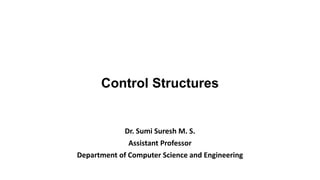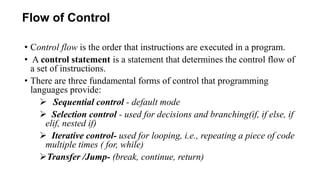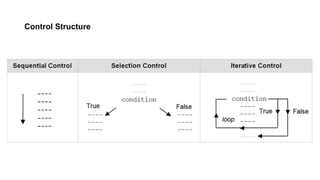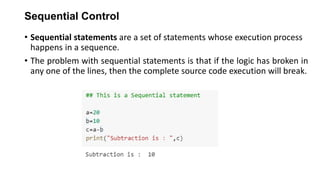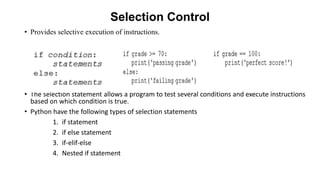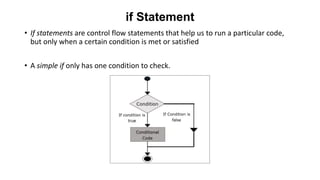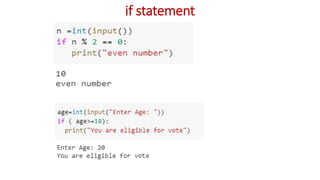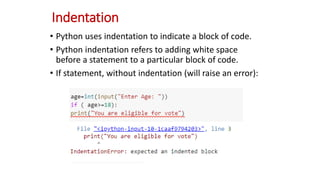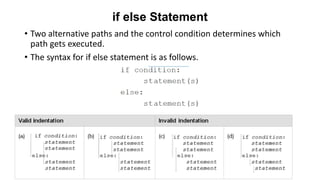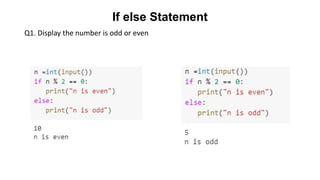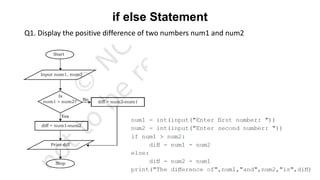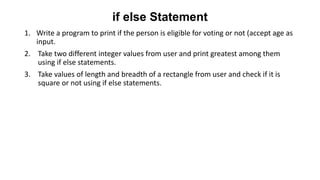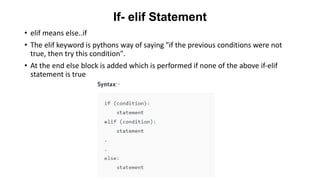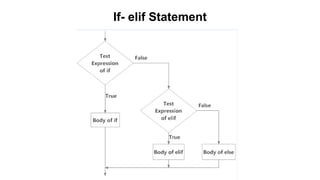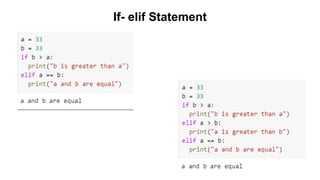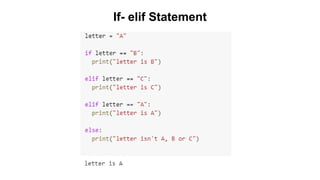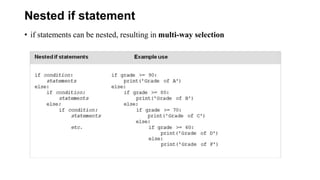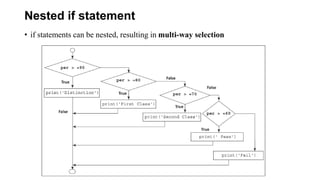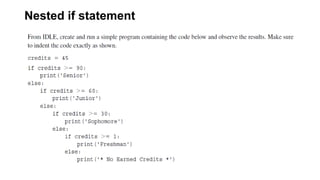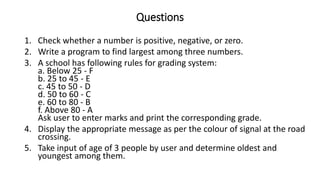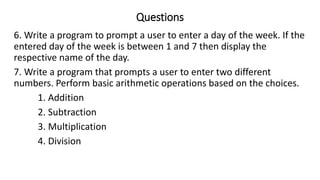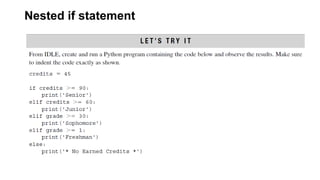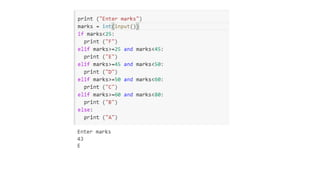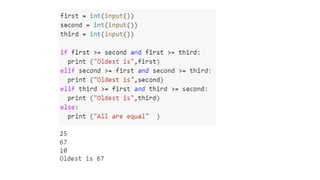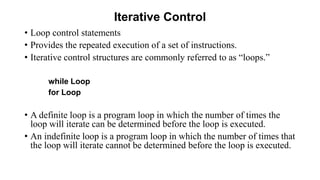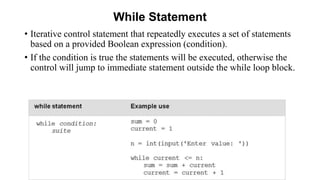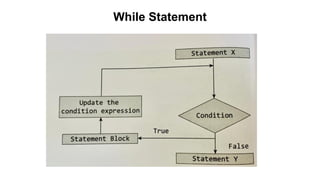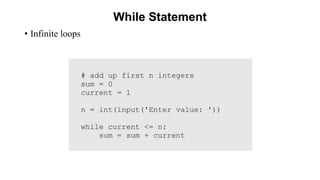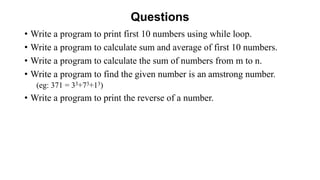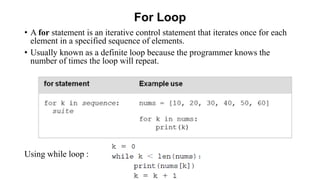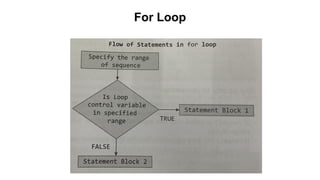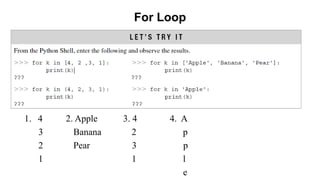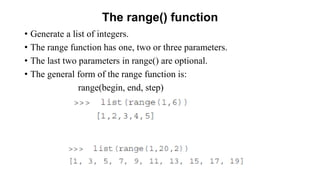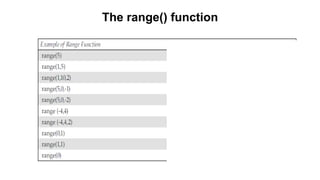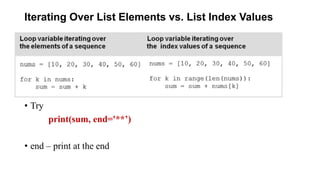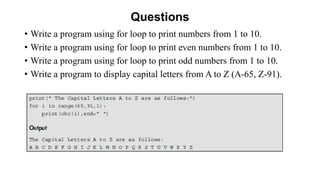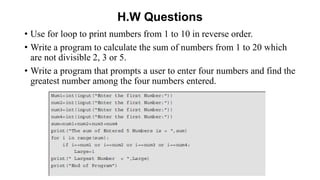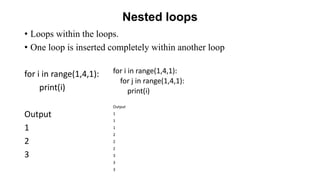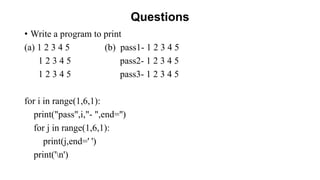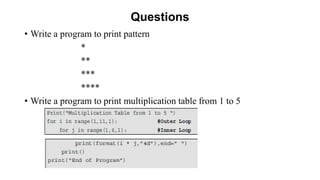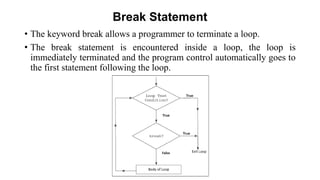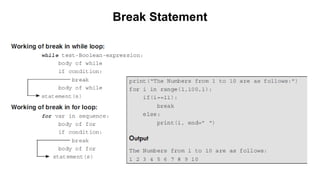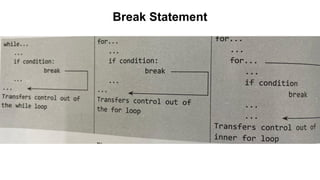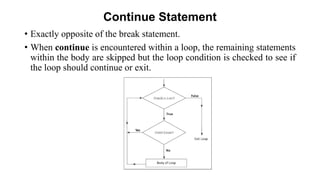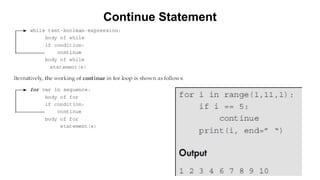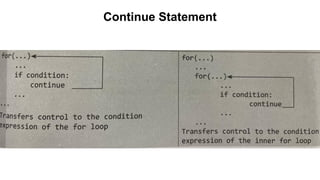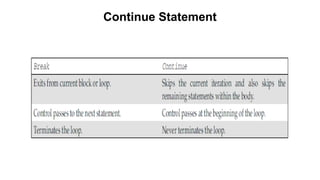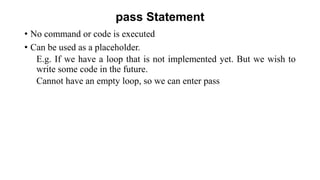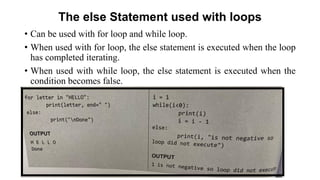1 de 49
Anúncio

### Slide 6_Control Structures.pdf

1. Control Structures Dr. Sumi Suresh M. S. Assistant Professor Department of Computer Science and Engineering
2. Flow of Control • Control flow is the order that instructions are executed in a program. • A control statement is a statement that determines the control flow of a set of instructions. • There are three fundamental forms of control that programming languages provide: ➢ Sequential control - default mode ➢ Selection control - used for decisions and branching(if, if else, if elif, nested if) ➢ Iterative control- used for looping, i.e., repeating a piece of code multiple times ( for, while) ➢Transfer /Jump- (break, continue, return)
3. Control Structure
4. Sequential Control • Sequential statements are a set of statements whose execution process happens in a sequence. • The problem with sequential statements is that if the logic has broken in any one of the lines, then the complete source code execution will break.
5. Selection Control • Provides selective execution of instructions. • The selection statement allows a program to test several conditions and execute instructions based on which condition is true. • Python have the following types of selection statements 1. if statement 2. if else statement 3. if-elif-else 4. Nested if statement
6. if Statement • If statements are control flow statements that help us to run a particular code, but only when a certain condition is met or satisfied • A simple if only has one condition to check.
7. if statement
8. Indentation • Python uses indentation to indicate a block of code. • Python indentation refers to adding white space before a statement to a particular block of code. • If statement, without indentation (will raise an error):
9. if else Statement • Two alternative paths and the control condition determines which path gets executed. • The syntax for if else statement is as follows.
10. If else Statement Q1. Display the number is odd or even
11. if else Statement Q1. Display the positive difference of two numbers num1 and num2
12. if else Statement 1. Write a program to print if the person is eligible for voting or not (accept age as input. 2. Take two different integer values from user and print greatest among them using if else statements. 3. Take values of length and breadth of a rectangle from user and check if it is square or not using if else statements.
13. If- elif Statement • elif means else..if • The elif keyword is pythons way of saying "if the previous conditions were not true, then try this condition". • At the end else block is added which is performed if none of the above if-elif statement is true
14. If- elif Statement
15. If- elif Statement
16. If- elif Statement
17. Nested if statement • if statements can be nested, resulting in multi-way selection
18. Nested if statement • if statements can be nested, resulting in multi-way selection
19. Nested if statement
20. Questions 1. Check whether a number is positive, negative, or zero. 2. Write a program to find largest among three numbers. 3. A school has following rules for grading system: a. Below 25 - F b. 25 to 45 - E c. 45 to 50 - D d. 50 to 60 - C e. 60 to 80 - B f. Above 80 - A Ask user to enter marks and print the corresponding grade. 4. Display the appropriate message as per the colour of signal at the road crossing. 5. Take input of age of 3 people by user and determine oldest and youngest among them.
21. Questions 6. Write a program to prompt a user to enter a day of the week. If the entered day of the week is between 1 and 7 then display the respective name of the day. 7. Write a program that prompts a user to enter two different numbers. Perform basic arithmetic operations based on the choices. 1. Addition 2. Subtraction 3. Multiplication 4. Division
22. Nested if statement
23. Iterative Control • Loop control statements • Provides the repeated execution of a set of instructions. • Iterative control structures are commonly referred to as “loops.” while Loop for Loop • A definite loop is a program loop in which the number of times the loop will iterate can be determined before the loop is executed. • An indefinite loop is a program loop in which the number of times that the loop will iterate cannot be determined before the loop is executed.
24. While Statement • Iterative control statement that repeatedly executes a set of statements based on a provided Boolean expression (condition). • If the condition is true the statements will be executed, otherwise the control will jump to immediate statement outside the while loop block.
25. While Statement
26. While Statement • Infinite loops
27. Questions • Write a program to print first 10 numbers using while loop. • Write a program to calculate sum and average of first 10 numbers. • Write a program to calculate the sum of numbers from m to n. • Write a program to find the given number is an amstrong number. (eg: 371 = 33+73+13) • Write a program to print the reverse of a number.
28. For Loop • A for statement is an iterative control statement that iterates once for each element in a specified sequence of elements. • Usually known as a definite loop because the programmer knows the number of times the loop will repeat. Using while loop :
29. For Loop
30. For Loop 1. 4 2. Apple 3. 4 4. A 3 Banana 2 p 2 Pear 3 p 1 1 l e
31. The range() function • Generate a list of integers. • The range function has one, two or three parameters. • The last two parameters in range() are optional. • The general form of the range function is: range(begin, end, step)
32. The range() function
33. Iterating Over List Elements vs. List Index Values • Try print(sum, end='**’) • end – print at the end
34. Questions • Write a program using for loop to print numbers from 1 to 10. • Write a program using for loop to print even numbers from 1 to 10. • Write a program using for loop to print odd numbers from 1 to 10. • Write a program to display capital letters from A to Z (A-65, Z-91).
35. H.W Questions • Use for loop to print numbers from 1 to 10 in reverse order. • Write a program to calculate the sum of numbers from 1 to 20 which are not divisible 2, 3 or 5. • Write a program that prompts a user to enter four numbers and find the greatest number among the four numbers entered.
36. Nested loops • Loops within the loops. • One loop is inserted completely within another loop for i in range(1,4,1): print(i) Output 1 2 3 for i in range(1,4,1): for j in range(1,4,1): print(i) Output 1 1 1 2 2 2 3 3 3
37. Questions • Write a program to print (a) 1 2 3 4 5 (b) pass1- 1 2 3 4 5 1 2 3 4 5 pass2- 1 2 3 4 5 1 2 3 4 5 pass3- 1 2 3 4 5 for i in range(1,6,1): print("pass",i,"- ",end='') for j in range(1,6,1): print(j,end=' ') print('n')
38. Questions • Write a program to print pattern * ** *** **** • Write a program to print multiplication table from 1 to 5
39. Break Statement • The keyword break allows a programmer to terminate a loop. • The break statement is encountered inside a loop, the loop is immediately terminated and the program control automatically goes to the first statement following the loop.
40. Break Statement
41. Break Statement
42. Continue Statement • Exactly opposite of the break statement. • When continue is encountered within a loop, the remaining statements within the body are skipped but the loop condition is checked to see if the loop should continue or exit.
43. Continue Statement
44. Continue Statement
45. Continue Statement
46. pass Statement • No command or code is executed • Can be used as a placeholder. E.g. If we have a loop that is not implemented yet. But we wish to write some code in the future. Cannot have an empty loop, so we can enter pass
47. The else Statement used with loops • Can be used with for loop and while loop. • When used with for loop, the else statement is executed when the loop has completed iterating. • When used with while loop, the else statement is executed when the condition becomes false.
Anúncio